## MTSO June NewsThere is excitement in the air as we are all making plans for summer! Canada Day will soon be here, and we are also celebrating our Canadian made products. Feel confident knowing you're using only the best quality products with your clients.

Looking for upgrades to your clinic or home office? Call us at 780-440-1818 for suggestions on how to update your workspace with an affordable electric table, a sweet aromatherapy program, or new sheets.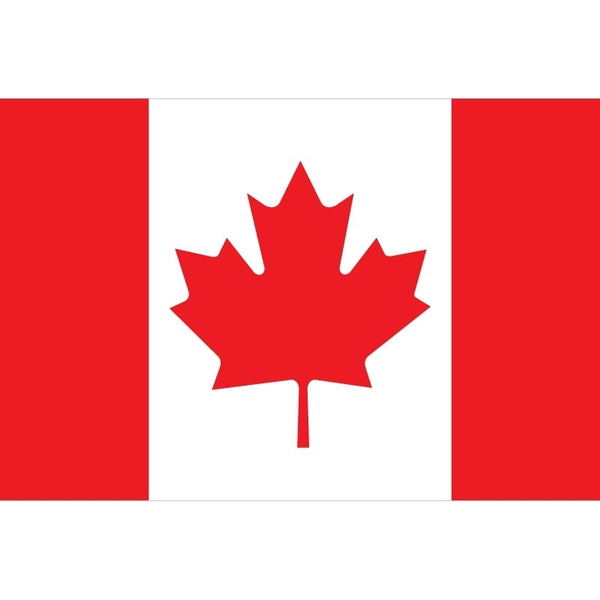We post the flag on all our Canadian products in our online store.

 table div table+table+table+table+table div table{width:100%;padding:0}table div table+table+table+table+table div table img{width:96.23%;padding:0;float:none}table div table+table+table+table+table div table td{width:100%;padding:0 1.88% 18px}/* styles *//* styles */

# Stock up on our Canadian Made linens - designed by RMTs for RMTs.

These beautiful locally-made massage linens are perfectly created especially for your professional massage and bodywork practice. Mix and match the colours, or work with pure white. Our customers have come to depend on our well-made sheets and cradle covers. Whether soft flannelette or cool crisp poly-cotton, our massage linens are known to be the best in the country. We are proud to ship our locally-produced linens throughout Canada.

 table div table+table+table+table+table+table+table+table+table div table{width:100%;padding:0}table div table+table+table+table+table+table+table+table+table div table img{width:96.23%;padding:0;float:none}table div table+table+table+table+table+table+table+table+table div table td{width:100%;padding:0 1.88% 18px}/* styles */# LIQUID SUNSHINE™ MASSAGE OIL

An ideal natural blend of 3 oils providing nourishing skin care for northern climates. This nut-free, unscented oil is suitable for a wide variety of massage applications.

▪ Sunflower Seed oil, high in vitamins A, B, D & E, minerals & unsaturated fatty acids, is light and easily absorbed.
▪ Fractionated Coconut oil, also high in vitamin E, is a smooth, emollient, easily absorbed oil, providing consistent glide and excellent transport for Aromatherapy oils.
▪ Jojoba oil being rich in vitamins A, D & E, is also soothing and emollient.
▪ The effect is that both client and therapist finish with smooth moisturized skin.
▪ The inclusion of a natural water dispersant assures easy laundering.
 ▪ Sunflower Seed oil, high in vitamins A, B, D & E, minerals & unsaturated fatty acids, is light and easily absorbed.
 ▪ Fractionated Coconut oil, also high in vitamin E, is a smooth, emollient, easily absorbed oil, providing consistent glide and excellent transport for Aromatherapy oils.
 ▪ Jojoba oil being rich in vitamins A, D & E, is also soothing and emollient.
 ▪ The effect is that both client and therapist finish with smooth moisturized skin.
 ▪ The inclusion of a natural water dispersant assures easy laundering.

Available in 2 sizes: 1 litre & 4 litres

 table div table+table+table+table+table+table+table+table+table+table+table+table div table{width:100%;padding:0}table div table+table+table+table+table+table+table+table+table+table+table+table div table img{width:96.23%;padding:0;float:none}table div table+table+table+table+table+table+table+table+table+table+table+table div table td{width:100%;padding:0 1.88% 18px}/* styles */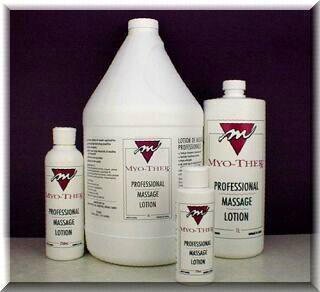MYO-THER SIGNATURE LOTION 4 LITRES

# MYO-THER SIGNATURE LOTION 4 LITRES

\$64.95
A Superior lubricating lotion developed by a Registered Massage Therapist for use by Professional Healthcare Practitioners. This product provides a long lasting, friction reducing lubricant for the skin that can be easily washed off.

Myo-ther applies smoothly to the skin, clarifying and spreading evenly with body heat. The formulation contains millions of tiny droplets that remain on the surface of the skin for long lasting lubrication, eliminating the need for constant re-applications.

 table div table+table+table+table+table+table+table+table+table+table+table+table+table+table+table div table{width:100%;padding:0}table div table+table+table+table+table+table+table+table+table+table+table+table+table+table+table div table img{width:96.23%;padding:0;float:none}table div table+table+table+table+table+table+table+table+table+table+table+table+table+table+table div table td{width:100%;padding:0 1.88% 18px}/* styles */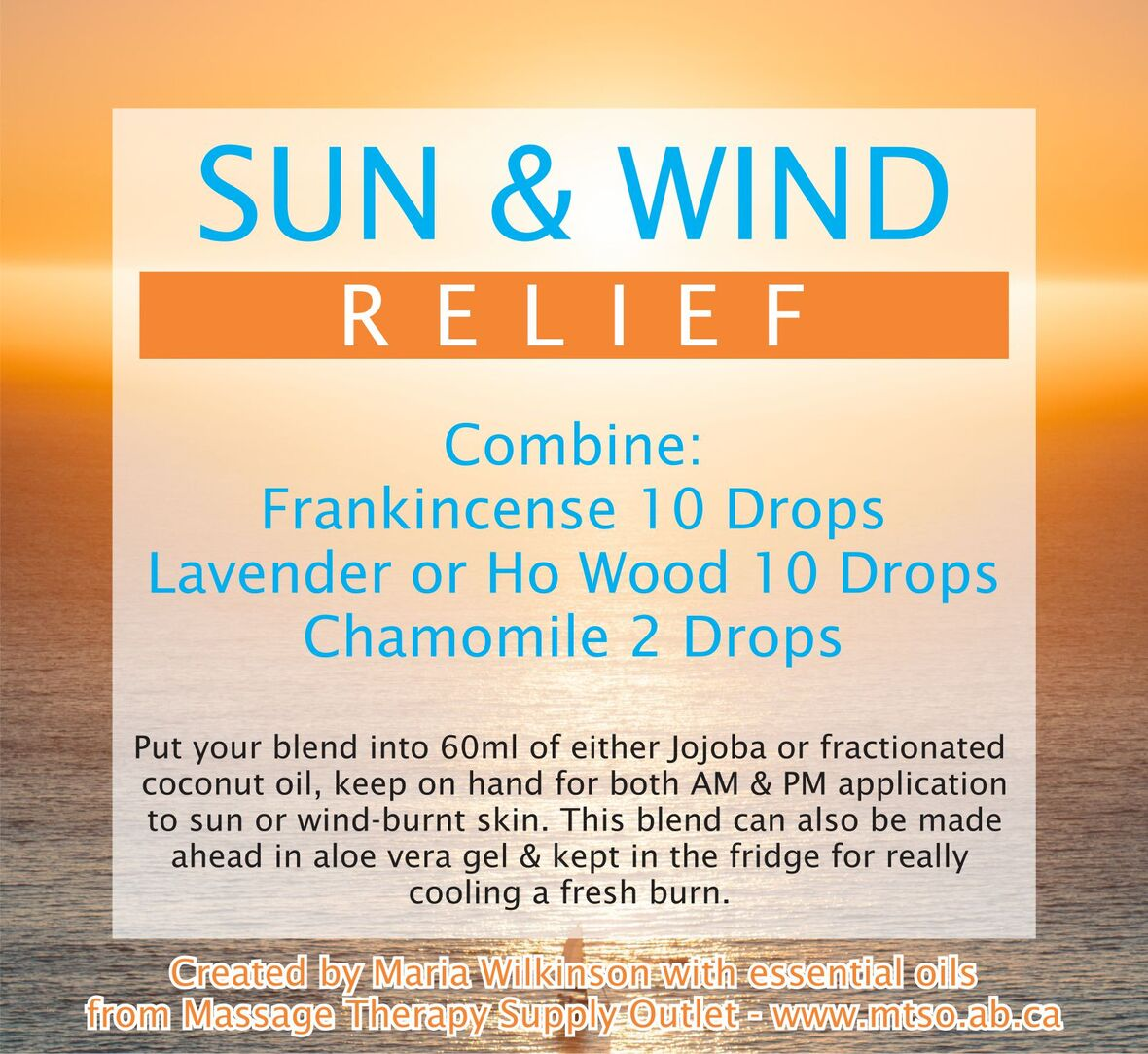# MAKE YOUR OWN! SUN AND WIND RELIEF ESSENTIAL OIL BLEND

 /* styles */ This very easy and effective recipe combines Frankincense (10 drops), Lavender or Ho Wood (10 drops) and Chamomile (2 drops). Put your blend into 60ml of either Jojoba or Fractionated coconut oil, and keep on hand for both morning and evening application to sun or wind-burnt skin. This blend can also be made ahead in aloe vera gel and kept in the fridge to really cool a fresh burn. It's a great blend to have on hand for your clients, and kids as well.
 table div table+table+table+table+table+table+table+table+table+table+table+table+table+table+table+table+table+table+table+table div table{width:100%;padding:0}table div table+table+table+table+table+table+table+table+table+table+table+table+table+table+table+table+table+table+table+table div table img{width:96.23%;padding:0;float:none}table div table+table+table+table+table+table+table+table+table+table+table+table+table+table+table+table+table+table+table+table div table td{width:100%;padding:0 1.88% 18px}/* styles */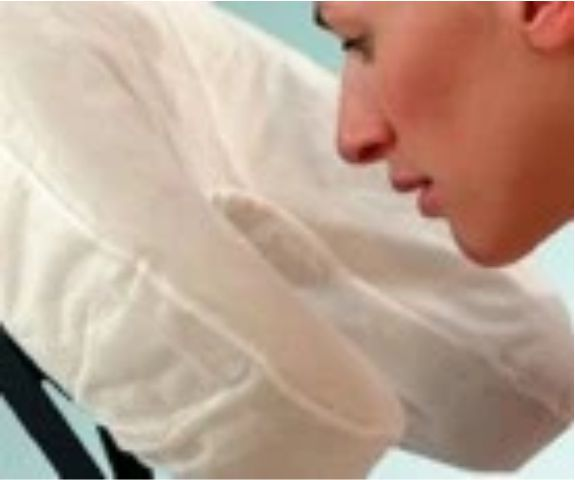Fitted Disposable Face Cradle Covers

# FITTED DISPOSABLE FACE CRADLE COVERS

Made from soft, 25 gsm spunbound fabric, this new and innovative design provides a comfortable, sanitary and disposable barrier for your clients and an easy changeover for between client protection.

▪ Fits all crescent-shaped face rests
▪ Stays put throughout your treatment
▪ Great for table and seated massage
▪ Box of 50
▪ Latex Free

 table div table+table+table+table+table+table+table+table+table+table+table+table+table+table+table+table+table+table+table+table+table+table+table div table{width:100%;padding:0}table div table+table+table+table+table+table+table+table+table+table+table+table+table+table+table+table+table+table+table+table+table+table+table div table img{width:96.23%;padding:0;float:none}table div table+table+table+table+table+table+table+table+table+table+table+table+table+table+table+table+table+table+table+table+table+table+table div table td{width:100%;padding:0 1.88% 18px}/* styles */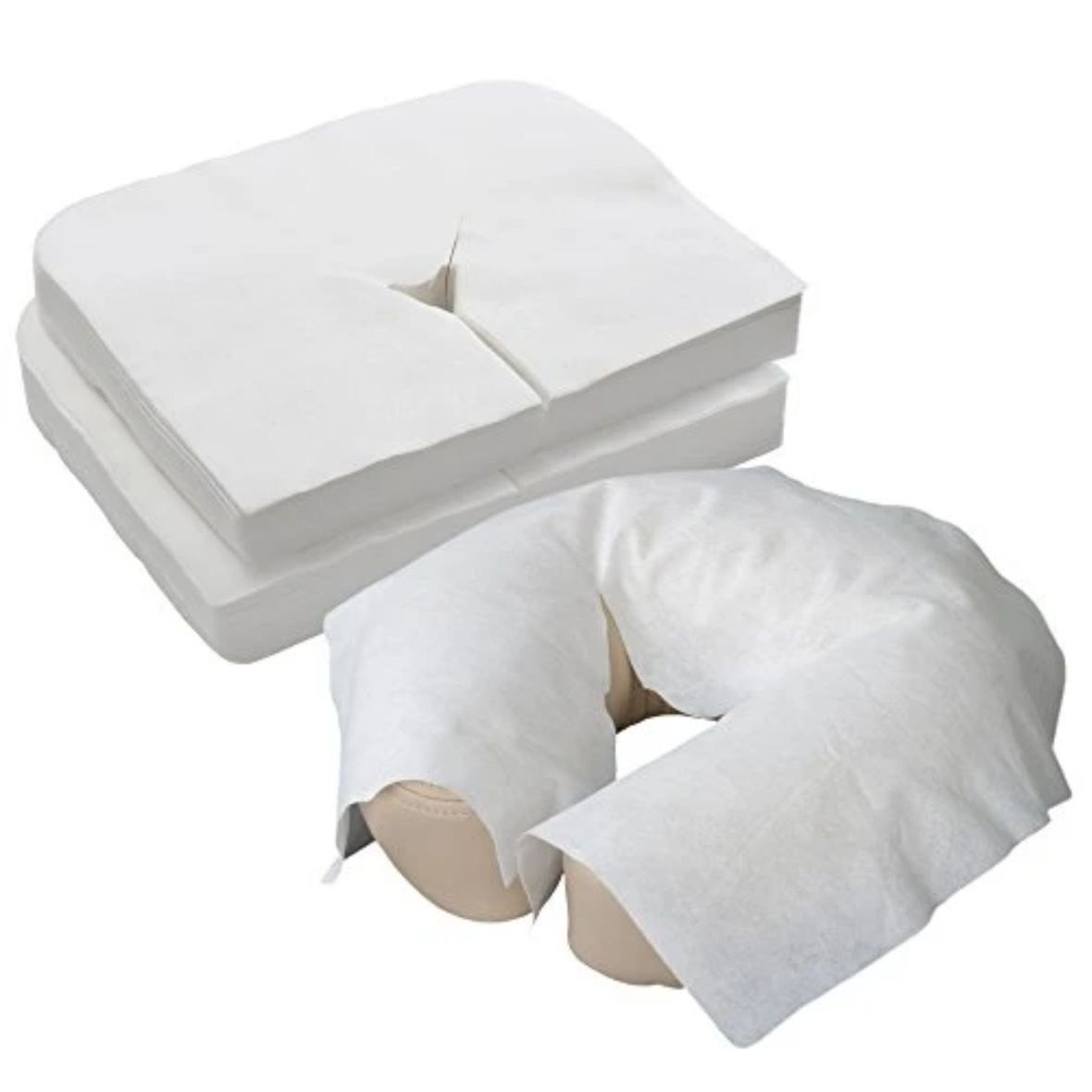Flat Disposable Face Cradle Covers

# FLAT DISPOSABLE FACE CRADLE COVERS

Flat soft disposable face cradle covers package of 100. Product stores easily in table and chair carry cases. Thick, yet soft and silky fabric conforms to the shape of your cushion. Feels like napped cotton. Flat face covers fit almost anything.

 table div table+table+table+table+table+table+table+table+table+table+table+table+table+table+table+table+table+table+table+table+table+table+table+table+table+table div table{width:100%;padding:0}table div table+table+table+table+table+table+table+table+table+table+table+table+table+table+table+table+table+table+table+table+table+table+table+table+table+table div table img{width:96.23%;padding:0;float:none}table div table+table+table+table+table+table+table+table+table+table+table+table+table+table+table+table+table+table+table+table+table+table+table+table+table+table div table td{width:100%;padding:0 1.88% 18px}/* styles */table.module-27{width:95.85%;padding:0}table div table+table+table+table+table+table+table+table+table+table+table+table+table+table+table+table+table+table+table+table+table+table+table+table+table+table+table+table div table{width:95.85%;float:none;margin-left:auto;margin-right:auto;padding:0}table div table+table+table+table+table+table+table+table+table+table+table+table+table+table+table+table+table+table+table+table+table+table+table+table+table+table+table+table div table a{border:0 none;text-decoration:none}table div table+table+table+table+table+table+table+table+table+table+table+table+table+table+table+table+table+table+table+table+table+table+table+table+table+table+table+table div table img{width:100%!important;border:0 none;text-decoration:none}table div table+table+table+table+table+table+table+table+table+table+table+table+table+table+table+table+table+table+table+table+table+table+table+table+table+table+table+table div table td{width:100%;padding:0}/* styles */
 table div table+table+table+table+table+table+table+table+table+table+table+table+table+table+table+table+table+table+table+table+table+table+table+table+table+table+table+table+table+table div table{width:100%;padding:0}table div table+table+table+table+table+table+table+table+table+table+table+table+table+table+table+table+table+table+table+table+table+table+table+table+table+table+table+table+table+table div table img{width:96.23%;padding:0;float:none}table div table+table+table+table+table+table+table+table+table+table+table+table+table+table+table+table+table+table+table+table+table+table+table+table+table+table+table+table+table+table div table td{width:100%;padding:0 1.88% 18px}/* styles */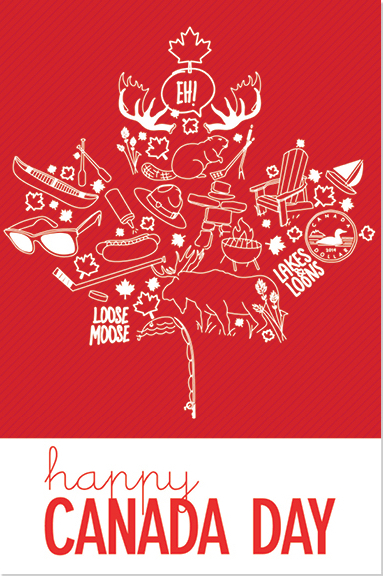# STORE CLOSED for Canada Day

We will be closed on Thursday, July 1 for Canada Day. We'll be open regular hours 10am to 5pm on Friday, July 2. Our online store is 24/7 www.mtso.ab.ca

 table div table+table+table+table+table+table+table+table+table+table+table+table+table+table+table+table+table+table+table+table+table+table+table+table+table+table+table+table+table+table+table+table div table{width:100%;padding:0}table div table+table+table+table+table+table+table+table+table+table+table+table+table+table+table+table+table+table+table+table+table+table+table+table+table+table+table+table+table+table+table+table div table img{width:96.23%;padding:0;float:none}table div table+table+table+table+table+table+table+table+table+table+table+table+table+table+table+table+table+table+table+table+table+table+table+table+table+table+table+table+table+table+table+table div table td{width:100%;padding:0 1.88% 18px}/* styles */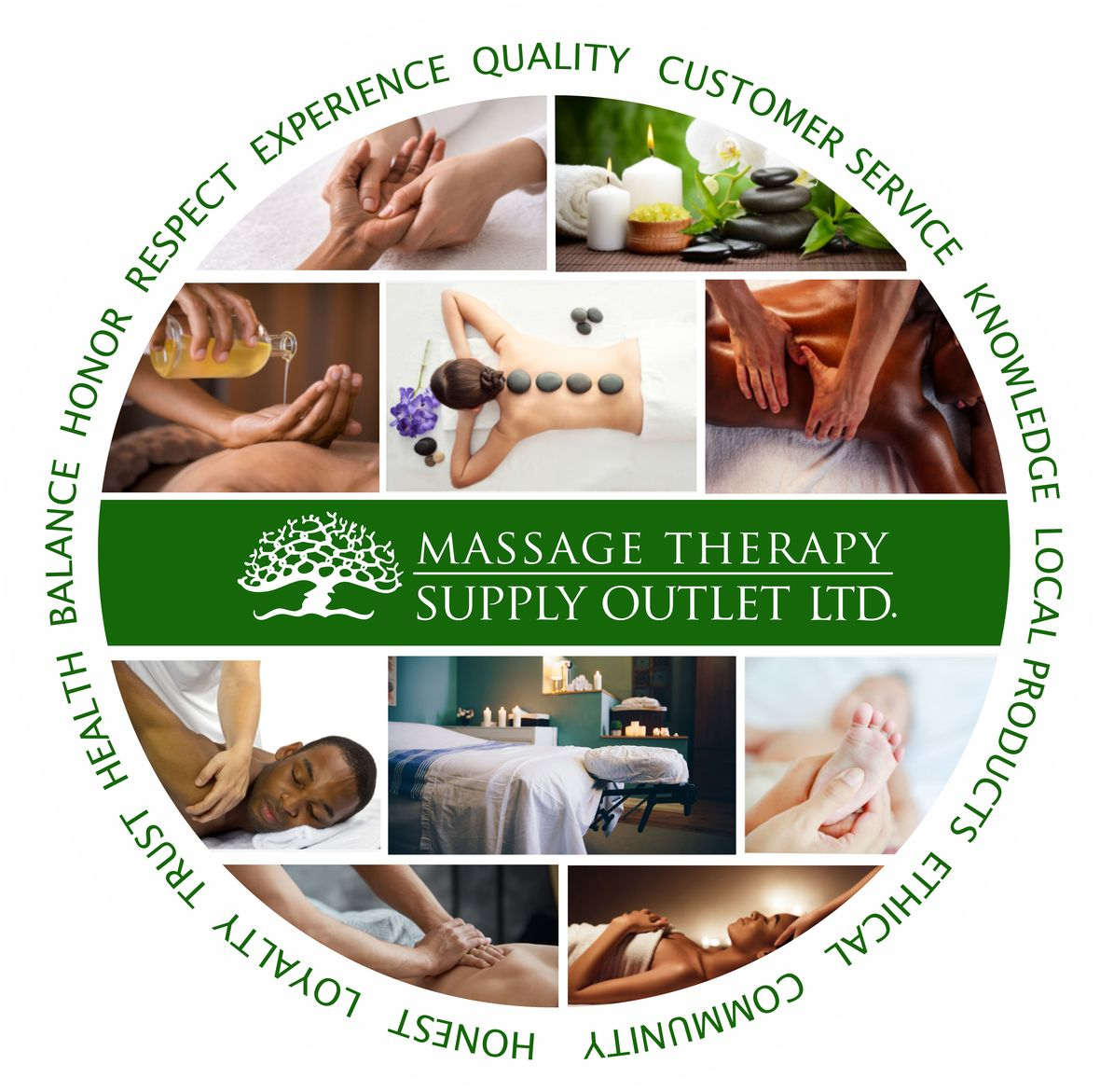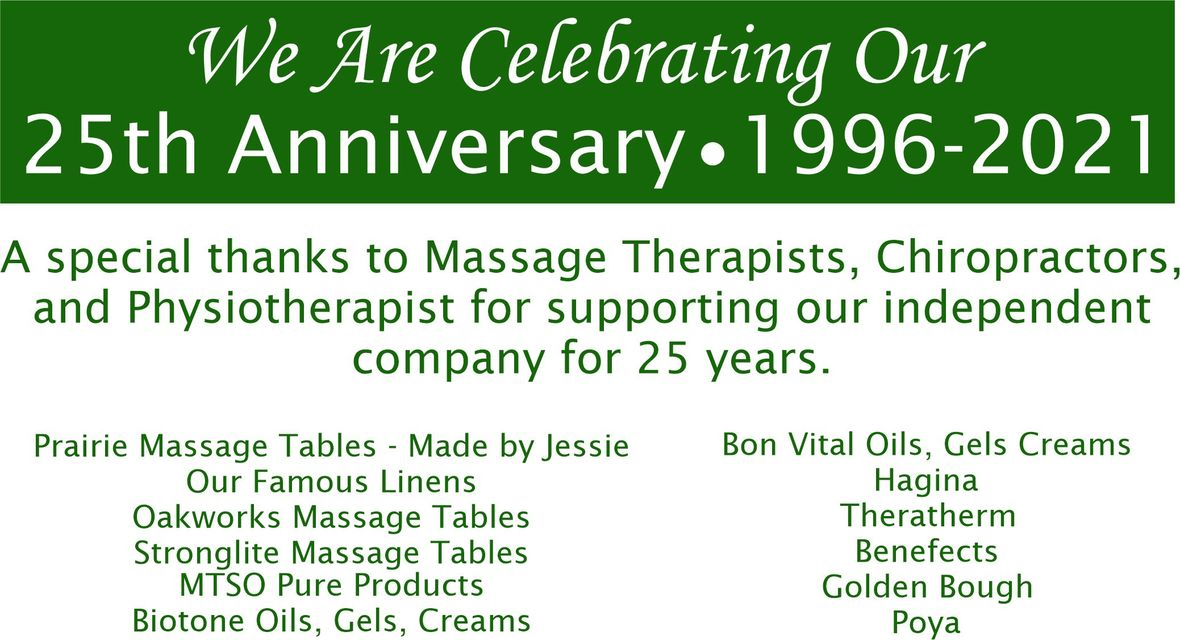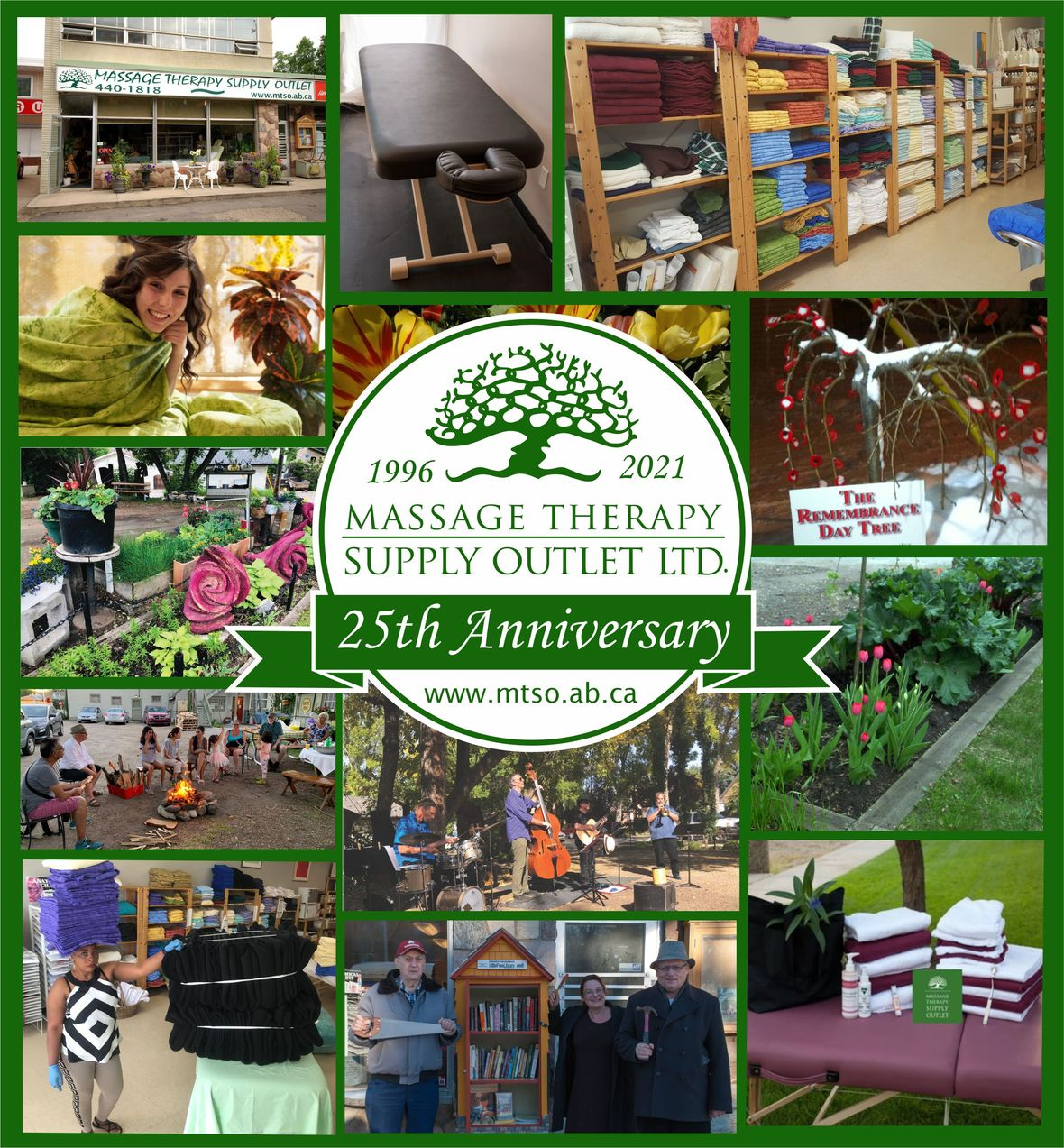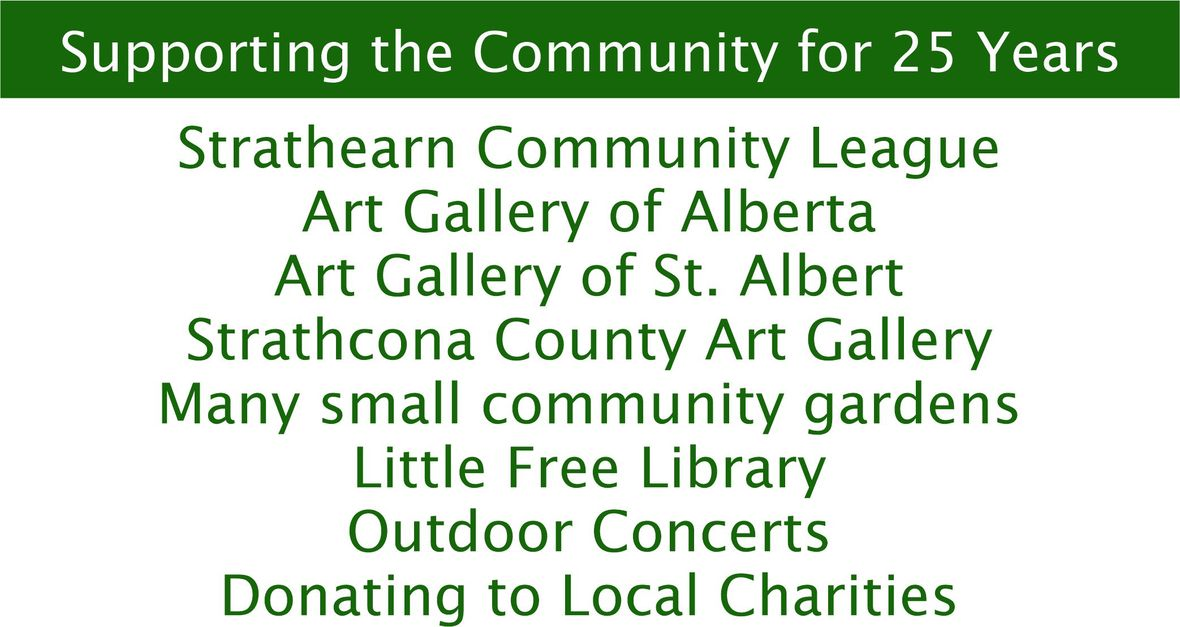table div table+table+table+table+table+table+table+table+table+table+table+table+table+table+table+table+table+table+table+table+table+table+table+table+table+table+table+table+table+table+table+table+table+table+table+table+table div table{width:100%;padding:0}table div table+table+table+table+table+table+table+table+table+table+table+table+table+table+table+table+table+table+table+table+table+table+table+table+table+table+table+table+table+table+table+table+table+table+table+table+table div table img{width:96.23%;padding:0;float:none}table div table+table+table+table+table+table+table+table+table+table+table+table+table+table+table+table+table+table+table+table+table+table+table+table+table+table+table+table+table+table+table+table+table+table+table+table+table div table td{width:100%;padding:0 1.88% 18px}/* styles */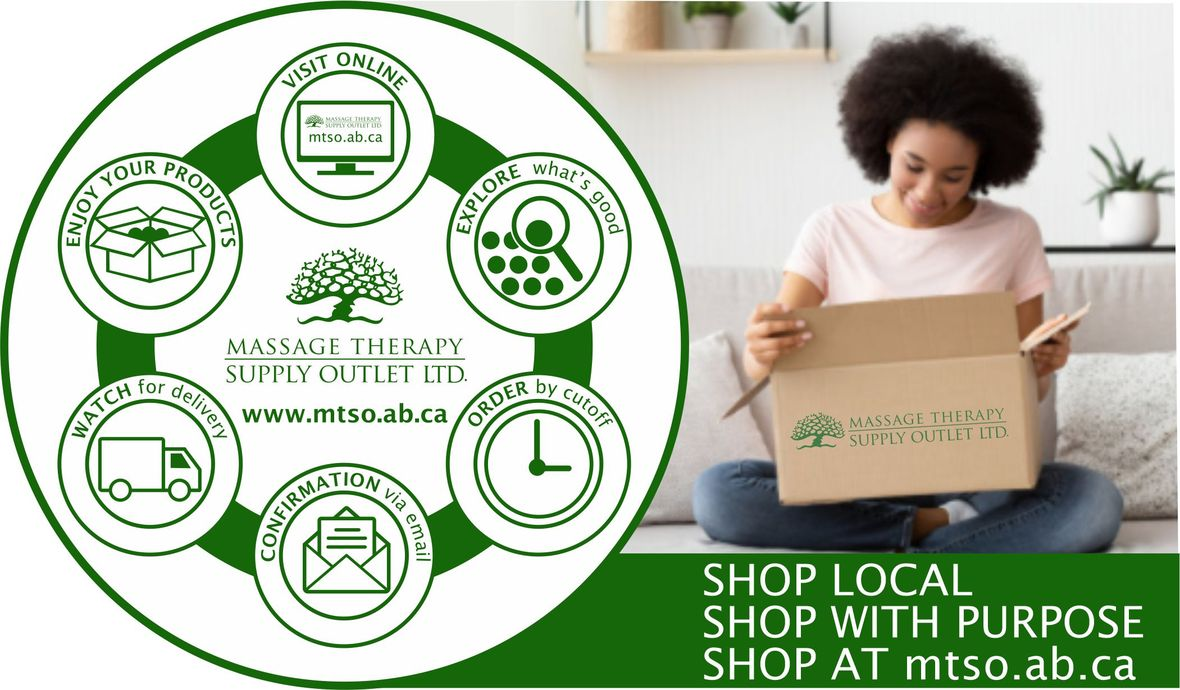# CURBSIDE PICKUP IS AVAILABLE

In addition to in-store shopping and shipping, curbside pick-up is also available. You can either phone-in your order or place order online. When placing your order online choose Local Pick Up at check out. We will contact you when your order is ready.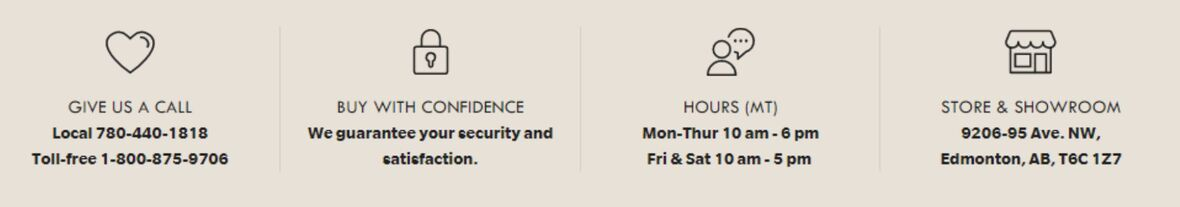/* styles */ Browse and buy from the extensive collection of quality products at our online store, always open, 24/7/365. Drop by our Edmonton store and showroom: 10:00 - 6:00 Mon-Thur, 10:00-5:00 Fri & Sat.
 Like   Tweet   Pin   in